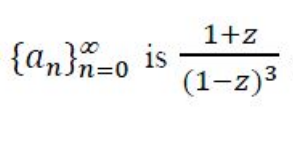# GATE | GATE-CS-2017 (Set 2) | Question 52

If the ordinary generating function of a sequence is:then a3-a0 is equal to:
(A) 8
(B) 10
(C) 15
(D) 20

Explanation: Given, ordinary generating function of an infinite sequence is:

Since, (1−z)−3 = 1+(3C1).z + (4C2).z2 + (5C3).z3 + … ∞

So, (1+z)(1−z)−3 = (1+z)∗(1+(3C1).z + (4C2).z2 + (5C3).z3 + … ∞)

Therefore, coefficient of z0 = a0 = 1,
and a0 = coefficient of z3 = a3 = 4C2 + 5C3 = 6 + 10 = 16.
Hence, a3 – a0 = 16 – 1 = 15

Alternative way –

```(z(1+z))/(1-z)3 = ∑r2.zr

=> (1+z)/(1-z)3 = 1/z(0.z0 + 1.z1 + 4.z2 + 9.z3 + 16.z4 + ... )

=> the sequence generated  = 1.z0, 4.z1, 9.z2, 16.z3, ...

=> a0 = 1; and a3 = 16
=> a3 - a0 = 16 - 1 = 15 ```

This explanation is contributed by Krit Verma.

My Personal Notes arrow_drop_up
Article Tags :

Be the First to upvote.

Please write to us at contribute@geeksforgeeks.org to report any issue with the above content.